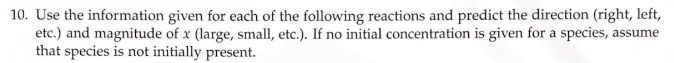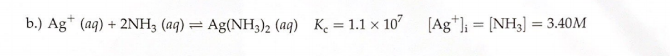# 10. Use the information given for each of the following reactions and predict the direction (right, left, etc.) and magnitude of x (large, small, etc). If no initial concentration is given for a species, assume that species is not initially present. b.) Ag^+ (aq) + 2NH3 (aq) ⇌ Ag(NH3)2 (aq) Kc = 1.1 X 10^7 [Ag^+]i = [NH3] = 3.40M